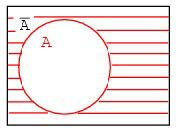## Pages

### Logical Operators : NOT OperatorIn boolean algebra any variable can have either of the two values, 0 or 1. And in terms of digital circuits it can be either of two values, LOW or HIGH. Like 0 and 1, LOW and HIGH are complements of each other. The NOT operator can be used to provide the complements of values (0,1 ; LOW, HIGH). It is a unary operator. It operates on single variable. It can be written as a bar above a variable ā or by the symbol such as a'. If a' is 1, then a= 0. Complementation or the NOT operation can be understood easily through Venn diagram.

In this diagram the circle represents a and the shaded portion of the rectangle represents ā , i.e, the complement of a.
If a=1 then ā = 0,
If a = True then ā = False.
The truth table for the NOT operator is
a            ā
0                  1
1                  0